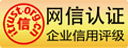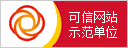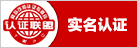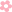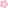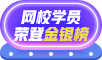APP下载# Vlookup函数公式大全，收藏这一篇就够了！

Vlookup函数是Excel中的一个纵向查找函数，它在工作中有着广泛应用，例如可以用来核对数据，多个表格之间快速导入数据等函数功能。下面小编为您整理了Vlookup函数所有公式，涵盖了一般查找、从左至右查找、查找公式复制、区间查找、模糊查找等等12个公式，赶快收藏起来吧！1、一般查找

【例1】如下图所示，根据姓名查找职位。

=VLOOKUP(B9,B1:E6,4,0)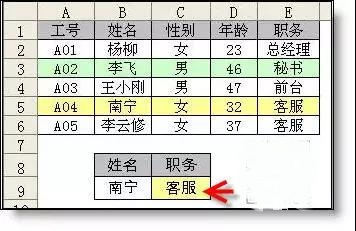2、从左至右查找

【例2】如下图所示，要求根据姓名查找工号。

=VLOOKUP(B9,IF({1,0},B2:B6,A2:A6),2,0)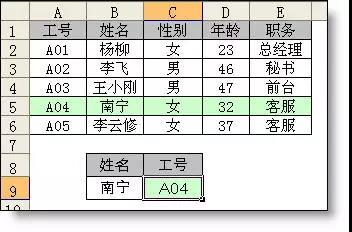3、查找公式复制

【例3】在表二中根据姓名从上表中查询对应信息。

=VLOOKUP(\$A13,\$B\$2:\$F\$8,COLUMN(B1),0)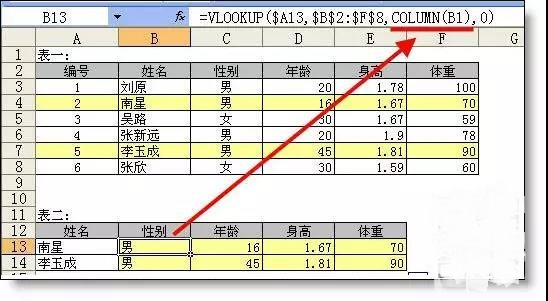4、区间查找

【例4】如下图所示,要求根据左表的提成比率表，在右表中根据销售额在G列查找适用的比率。

=VLOOKUP(F3,\$B\$3:\$C\$11,2)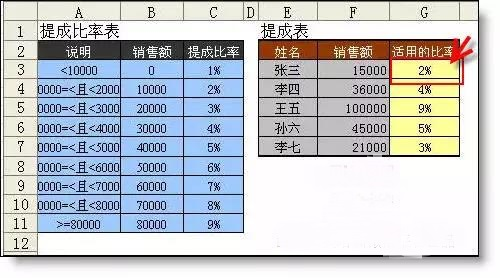5、模糊查找

【例5】查找包含AAA名称的产品价格

=VLOOKUP("*"&A10&"*",A2:B6,2,0)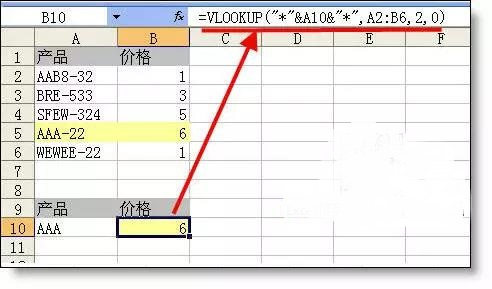6、多项查找

【例6】从下表中同时查找“李飞”和“南宁”的年龄 ，并返回最小的。

=MIN(VLOOKUP(T(IF(1,{"李飞","南宁"})),A2:C6,3,0))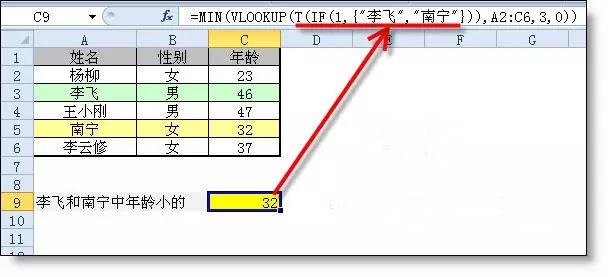7、隔任意列求和

{=SUM(VLOOKUP(A2,A2:K2,ROW(1:6)*2-1,0))}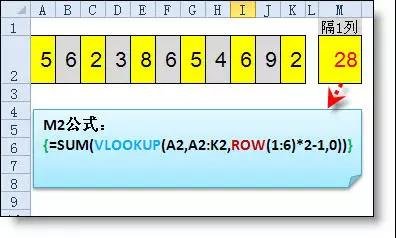{=SUM(VLOOKUP(A2,A2:K2,{2,3,5,6,8,9},0))}

{2,3,5,6,8,9}把要求和的列数放在大括号内，用逗号分隔。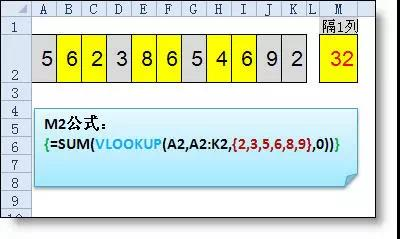8、多条件查找

【例8】如下图所示要求，同时根据姓名和工号查找职位。

{=VLOOKUP(B9&C9,IF({1,0},B2:B6&A2:A6,E2:E6),2,0)}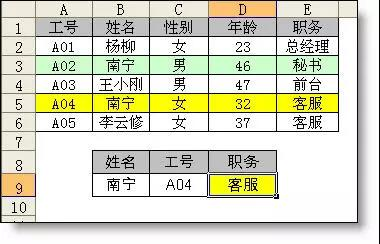9、多区域查找

【例9】根据不同的表从不同的区域查询

=VLOOKUP(B2,IF(A2="销售一部",A5:B9,D5:E9),2,0)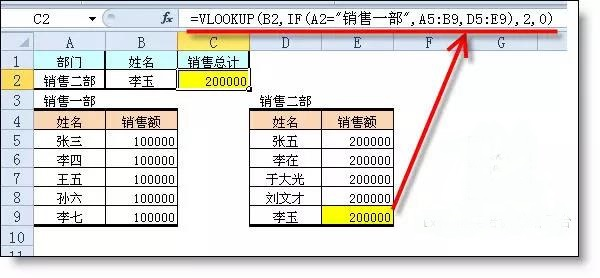10、多工作表查找

【例10】从各部门中查找员工的基本工资，在哪一个表中不一定。

=IFERROR(VLOOKUP(A2,服务!A:G,7,0),IFERROR(VLOOKUP(A2,人事!A:G,7,0),IFERROR(VLOOKUP(A2,综合!A:G,7,0),IFERROR(VLOOKUP(A2,财务!A:G,7,0),IFERROR(VLOOKUP(A2,销售!A:G,7,0),"无此人信息")))))

=VLOOKUP(A2,INDIRECT(LOOKUP(1,0/COUNTIF(INDIRECT({"销售";"服务";"人事";"综合";"财务"}&"!a:a"),A2),{"销售";"服务";"人事";"综合";"财务"})&"!a:g"),7,0)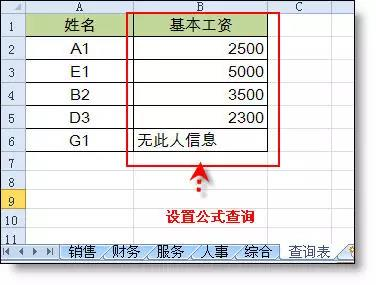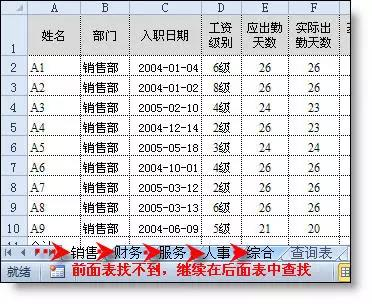11、一对多查找1

【例】根据产品查找相对应的所有供应商

A2 =B2&COUNTIF(B\$1:B2,B2)

B11=IFERROR(VLOOKUP(\$A11&COLUMN(A1),\$A:\$C,3,0),"")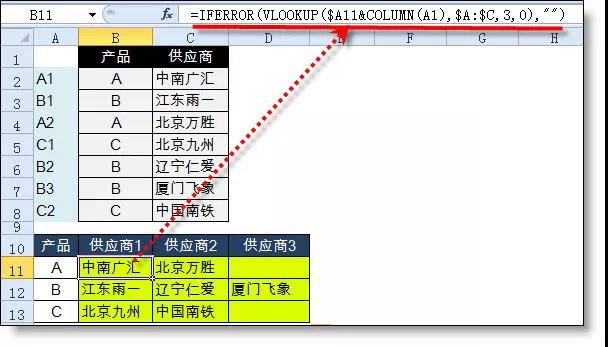12、一对多查找2

【例12】查找张三的所有消费记录

{=VLOOKUP(B\$9&ROW(A1),IF({1,0},\$B\$2:\$B\$6&COUNTIF(INDIRECT("b2:b"&ROW(\$2:\$6)),B\$9),\$C\$2:\$C\$6),2,)}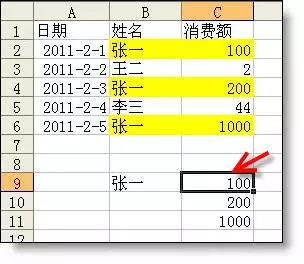## 实务学习指南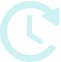距11月报税开始还有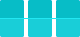天 能力测评 模拟实训 财税问答 资讯文章 财经法规 实务课程

## 学习方案

•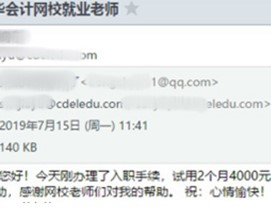37岁零基础转行做会计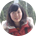会计新锐学员
•四十岁进入国企当财管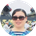财务主管训练营学员
•注会没考过也能进入事务所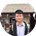事务所训练营学员
•考过中级的无经验小白成功就业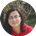会计新锐学员

## 限时免费资料

•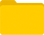财务表格

•纳税申报

•成本管理

•常用Excel

•实务资料库

## 加入实务学习战队APP下载